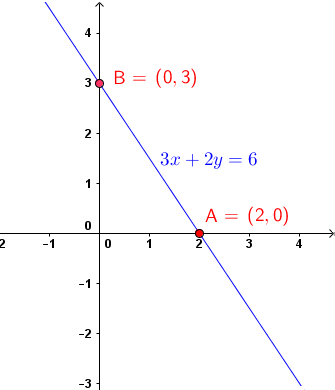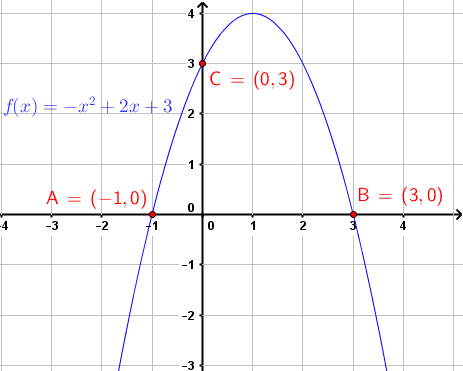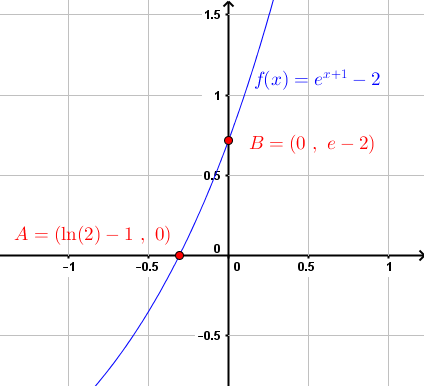# How to Find x and y Intercepts Of Graphs?

How to find the x and the y intercepts of graphs of functions and equations?
The x and y intercepts of a graph are points of intersection of the graph with the x axis and the y axis respectively. This is a tutorial with examples and detailed solutions on how to find these points.

## Example 1

Find the x and the y intercepts of the graph of function f defined by
f(x) = - 3 x + 9

Solution to Example 1

• Since a point on the y axis has x coordinate equal to zero, to find the y interecpt, we set x to zero and find the y coordinate which is f(0).
f(0) = -3(0) + 9 = 9
• A point on the x axis has y coordinate equal to 0, to find the x intercept, we set y = f(x) = 0 and solve for x
f(x) = -3 x + 9 = 0
• Solve for x.
x = 3
• The x and y intercepts of the graph of f are
x intercept: (3 , 0)
y intercept: (0 , 9)

## Example 2

Find the x and the y intercepts of the graph of the equation the circle given by
(x - 1) 2 + (y - 2) 2 = 16

Solution to Example 2

• To find y intercept: Set x = 0 in the equation and solve for y.
(0 - 1) 2 + (y - 2) 2 = 16
• Solve for y
1 + (y - 2) 2 = 16
(y - 2) 2 = 15
solutions: y1 = 2 + √(15) and y2 = 2 - √(15)
• To find x intercept: set y = 0 in the given equation and solve for x
(x - 1) 2 + (0 - 2) 2 = 16
solutions: x1 = 1 + √(12) and x2 = 1 - √(12)
• The x and y intercepts of the graph of the given equation are
x intercepts: A = (1 - √(12) , 0) and B = (1 + √(12) , 0)
y intercepts: C = (0 , 2 - √(15)) and D = (0 , 2 + √(15))
• The graph shown below is that of the given equation. Examine the x and y intercepts and compare to those calculated. Note that the x and y intercepts may be determined graphically.## Example 3

Calculate the x and the y intercepts of the graph of the linear equation given by
3x + 2y = 6

Solution to Example 3

• Set x = 0 in the given equation and find the y intercept.
3(0) + 2y = 6
• Solve for y
y = 3
• Set y = 0 and solve for x to find the x intercept
3 x + 2(0) = 6 , x = 2
• The x and y intercepts of the graph of the above equation are:
x intercepts: A = (2 , 0)
y intercepts: B = (0 , 3)
• The graph of the given equation is shown below. The x and y intercepts are those calculated above. Note that the x and y intercepts may be determined graphically.## Example 4

Calculate the x and the y intercepts of the graph of the quadratic function given by
f(x) = - x2 + 2 x + 3

Solution to Example 4

• Set x = 0 in the formula of the given function and calculate the y intercept which is equal to f(0).
y = f(0) = 3
• To find the x intercept: set y = f(x) = 0 and solve for x
- x2 + 2 x + 3 = 0
Solutions: x1 = -1 and x2 = 3
• The x and y intercepts of the graph of the above equation are:
x intercepts: A = (-1 , 0) and B = ( 3 , 0)
y intercepts: C = (0 , 3)
• The graph of the given function is shown below along with the x and y intercepts as calculated above.## Example 5

Determine the x and the y intercepts of the graph of the logarithmic function given by
f(x) = - ln(x + 1) - 2

Solution to Example 5

• Set x = 0 in the formula of the function and the y intercept is equal to f(0).
y = f(0) = - ln(0 + 1) - 2 = - 2
• Set y = f(x) = 0 and solve for x
- ln(x + 1) - 2 = 0
ln(x + 1) = -2
x + 1 = e-2
solution: x = - 1 + 1/e2
• The x and y intercepts of the graph of the above equation are:
x intercepts: A = (-1+1/e2 , 0)
y intercepts: B = ( 0 , - 2)
• The graph of the given function is shown below along with the x and y intercepts as calculated above.## Example 6

Calculate the x and the y intercepts of the graph of the exponential function given by
f(x) = ex + 1 - 2

Solution to Example 6

• The y intercept is equal to f(0).
y = f(0) = e0 + 1 - 2 = e - 2
• Set y = f(x) = 0 and solve for x
ex + 1 - 2 = 0
ex + 1 = 2
x + 1 = ln 2
solution: x = - 1 + ln 2
• The x and y intercepts of the graph of the above equation are:
x intercepts: A = (-1 + ln 2 , 0)
y intercepts: B = ( 0 , e - 2)
• The graph of the given function and its x and y intercepts are shown below.## Example 7

Calculate the x and the y intercepts of the graph of the rational function given by
f(x) = (x 2- x - 2) / (x 2 - x - 3)

Solution to Example 7

• The y intercept is equal to f(0).
y = f(0) = 2/3
• Set the numerator of f(x) equal to zero and solve for x to find the x intercepts
x 2 - x - 2 = 0
solution: x1 = - 1 and x2 = 2
• The x and y intercepts of the graph of the above function are:
x intercepts: A = (-1 , 0) and B = (2 , 0)
y intercepts: C = ( 0 , 2/3)
• The graph of the given function and the x and y intercepts are shown below.## Example 8

Calculate the x and the y intercepts of the graph of the sine function given by
f(x) = sin(x) + 1/2

Solution to Example 8

• The y intercept is equal to f(0).
y = f(0) = 1/2
• Set f(x) equal to zero and solve for x to fnd the x intercepts
sin(x) + 1/2 = 0 , sin(x) = -1/2
solution:Because of the periodicity of the sine function, there is an infinite number of x intercepts given by:
x1 = 7π/6 + 2kπ , k=0,~+mn~1 , ~+mn~2 , ...
x2 = 11π/6 + 2kπ , k=0,~+mn~1 , ~+mn~2 , ...
• Some of the x intercepts and the y intercept are:
x intercepts: A = (-π/6 , 0) , B = (7π/6 , 0) and C = (11π/6 , 0)
y intercepts: D = ( 0 , 1/2)
• The graph of the given function and the x and y intercepts are shown below.